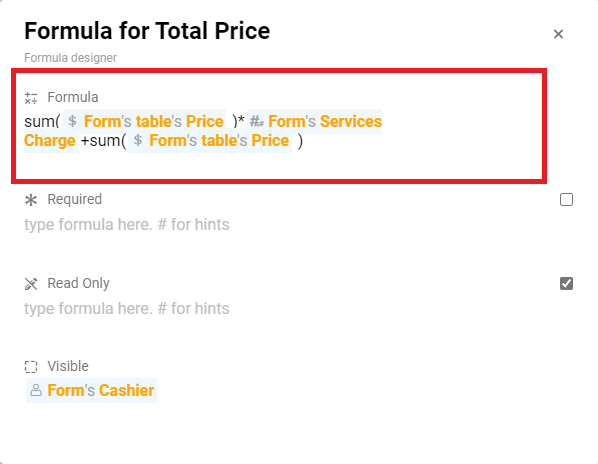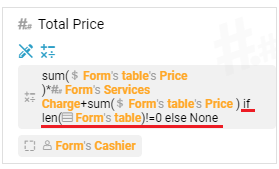# Formula to count a total of a column at table

To count the total of a column at a table, we can using the following formula:
Example Table:

Formula:With the formula above, it can already calculate the total of the table column “Price”. But you will faced a problem with this formula is, when you click “Done” and try to edit this entry again then remove a existed row. You will noticed that the “Total Price” information wont recalculate the Total of Price, so to force it to do that every time we edit the table. We need to add a if else condition into the formula:Which the formula condition above will force this “Total Price” information always recalculate the total when the numbers of table row had changed.

2 Likes